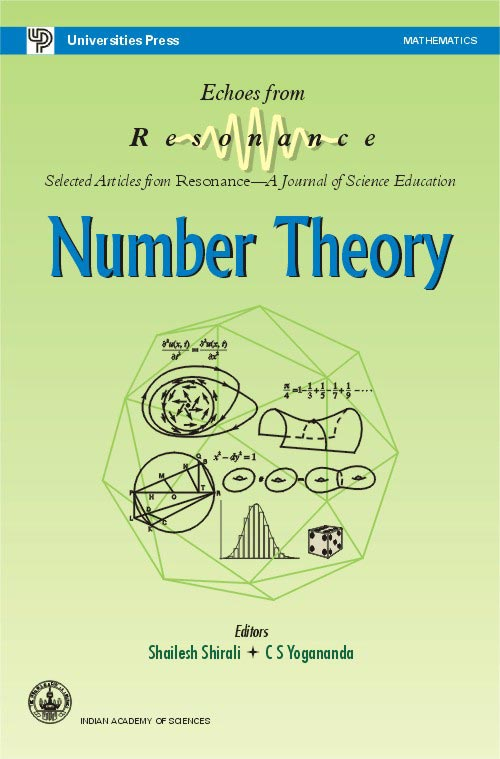## Number Theory

#### Price: 495.00

Number Theory has fascinated mathematicians from the most ancient of times. A remarkable feature of number theory is the fact that there is something in it for everyone—from puzzle enthusiasts, problem solvers and amateur mathematicians to professional scientists and technologists. This book offers the reader some articles in number theory that appeared in Resonance over the years 1996–2001. The articles included within form a varied lot, beginning with a puzzle, “find four positive integers such that the sum of any two is a square,” to an expository article on one of the great mathematical achievements of the 20th century—the proof of “Fermat’s Last Theorem”.

#### Contributors (Author(s), Editor(s), Translator(s), Illustrator(s) etc.)

Foreword
Preface

1. On Provability versus Consistency in Elementary Mathematics
SHAILESH A SHIRALI
2. To find Four Distinct Positive Integers such that the Sum of Any Two of them is a Square
S H ARAVIND
3. Bachet’s Problems
B BAGCHI
4. Mathematical Induction  --  An Impresario of the Infinite
B SURY
5. On the Infinitude of Prime Numbers  --  Euler’s Proof
SHAILESH A SHIRALI
6. On Fermat’s Two Squares Theorem
SHAILESH A SHIRALI
7. Fermat’s Two Squares Theorem Revisited
B BAGCHI
8. Factoring Fermat Numbers  --  A Unique Computational Experiment for Factoring F9
9. The Class Number Problem  --  Binary Quadratic Forms
RAJAT TANDON
10. The Class Number Problem  --  An Introduction to Algebraic Number Theory
RAJAT TANDON
11. Roots are Not Contained in Cyclotomic Fields
RAJAT TANDON
12. Die ganzenZahlen hat Gott gemacht  -- Polynomials with Integer Values
B SURY